Select Page

# Maths Three Dimensional Geometry CBSE 12 Science MCQ in English Solutions

Maths Three Dimensional Geometry CBSE 12 Science MCQ in English Solutions to enable students to get Solutions in a narrative video format for the specific question.

Expert Teacher provides Maths Three Dimensional Geometry CBSE 12 Science MCQ English Solutions. This video solution will be useful for students to understand how to write an answer in exam in order to score more marks. This teacher uses a narrative style for a question from Three Dimensional Geometry not only to explain the proper method of answering question, but deriving right answer too.

Please find the question below and view the Solution in a narrative video format.

Question:

Solution Video in English:

## Similar Questions from CBSE, 12th Science, Maths, Three Dimensional Geometry

Question 1 : Find the co-ordinates of the point where the line through the points (3, -4, -5) and (2, -3, 1) crosses the plane

Question 2 : If a line marks anglesand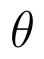with x, y and z-axis respectively, whereis acute, then find. (View Answer Video)

Question 3 : Find the distance of the point (3, 4, 5) from the plane x + y + z = 2 measured parallel to the line 2x = y = z. (View Answer Video)

Question 4 : If a line has the direction ratios -18, 12, -4, then what are its direction cosines? (View Answer Video)

Question 5 : Find the distance of the point (-1, -5, -10) from the point of intersection of the lineand the plane(View Answer Video)

### Integrals

Question 1 : Find :(View Answer Video)

Question 2 : Find the integral of the function. (View Answer Video)

Question 3 :  Find the integral of the function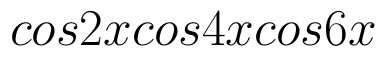. (View Answer Video)

Question 4 : Find :(View Answer Video)

Question 5 :

Evaluate the following definite integral :(View Answer Video)

### Determinant

Question 2 : If area of a triangle is 35 sq unit with vertices (2, -6), (5, 4) and (k, 4), then k is, (View Answer Video)

Question 3 : If A is a symmetric matrix, then? (View Answer Video)

Question 4 :  Using the properties of determinants, evaluate. (View Answer Video)

Question 5 :  Find the values of x, if. (View Answer Video)

### Probability

Question 1 : A couple has 2 children. Find the probability that both are boys, if it is known that one of them is a boy.  (View Answer Video)

Question 2 : A speaks truth in 75% of the cases, while B in 90% of the cases. In what percent of cases are they likely to contradict each other in stating the same fact? Do you think that statement of B is always true?     (View Answer Video)

Question 3 :  If E and F are independent events, then show that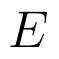and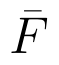Question 5 : If A and B are two events such that P(A)=0.3, P(B)=0.6 and P(B/A)=0.5, find P(A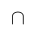B). (View Answer Video)